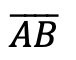# How to Construct an Equilateral Triangle?: Geometry

Happy Wednesday math peeps! This post introduces constructions by showing us how to construct an equilateral triangle by using a compass and a ruler. For anyone new to constructions, this is the perfect topic for art aficionados since there is more drawing than there is actual math.

## What is an Equilateral Triangle?

Equilateral Triangle: A triangle with three equal sides.  Not an easy one to forget, the equilateral triangle is super easy to construct given the right tools (compass+ ruler). Take a look below:

Now, for our Example:

## What’s Happening in this GIF?

1. Using a compass, measure out the distance of line segment.

2. With the compass on point A, draw an arc that has the same distance as.

3. With the compass on point B, draw an arc that has the same distance as.

4. Notice where the arcs intersect? Using a ruler, connect points A and B to the new point of intersection. This will create two new equal sides of our triangle!

Still got questions? No problem! Don’t hesitate to comment with any questions. Happy calculating! 🙂